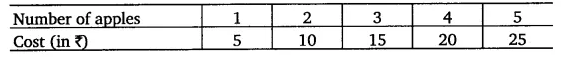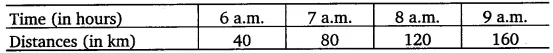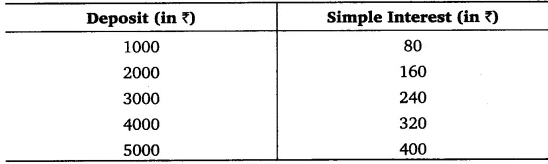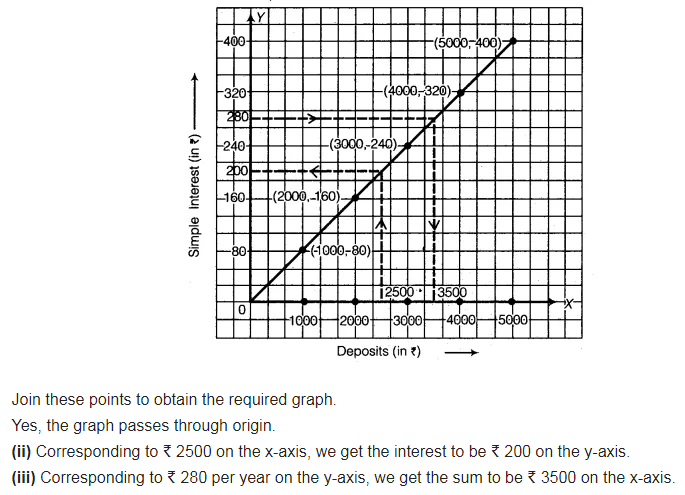# Draw the graphs for the following tables of values, with suitable scales on the axes

Draw the graphs for the following tables of values, with suitable scales on the axes.
(a) Cost of apples(b) Distance travelled by a car(i) How much distance did the car cover during the period 7.30 a.m. to 8 a.m.?
(ii) What was the time when the car had covered a distance of 100 km since it’s start? Interest on deposits for a year
( c) Interest on deposits for a year.(i) Does the graph pass through the origin?
(ii) Use the graph to find the interest on ( 2500 for a year.
(iii) To get an interest of? 280 per year, how much money should be deposited?